# Cube atop Icosahedron

Cube atop Icosahedron (cube || icosahedron), or K4.21, is one of Richard Klitzing's convex segmentochora, 4D CRF polytopes whose vertices lie on two parallel hyperplanes and which are orbiform (its vertices lie on a 4D sphere). It is constructed by placing a cube and an icosahedron in two appropriately-spaced parallel hyperplanes and taking their convex hull. Its surface consists of a cube, an icosahedron, 6 triangular prisms, 8 tetrahedra, and 12 square pyramids, for a total of 28 cells, 74 polygons (56 triangles, 18 squares), 66 edges, and 20 vertices.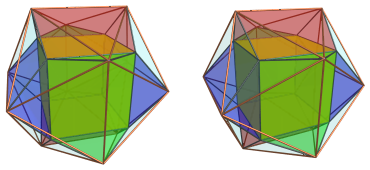K4.21 is a special case of a convex segmentochoron where the two generating polyhedra are taken from two different symmetry groups: the cube / octahedral group and the icosahedral group. This is unusual since the requirement for all edges to be equal length generally restricts viable constructions to those that involve two polyhedra from the same symmetry group. As such, K4.21 can be considered one of the crown jewels among the CRF polychora.

## Structure

We shall explore the structure of K4.21 using its parallel projections into 3D.

### The Near Side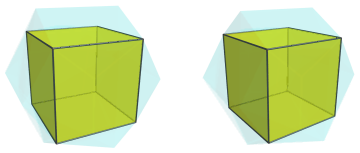The above image shows the cubical cell on the top of the polychoron. Its 6 square faces are joined to 6 triangular prisms: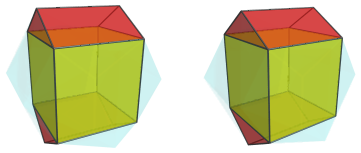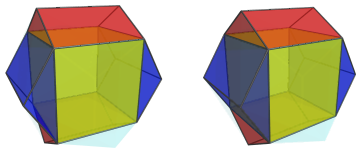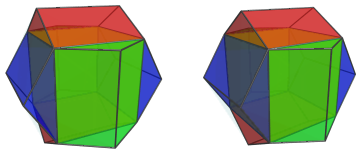These triangular prisms look squished because of foreshortening due to the projection; they lie at an angle to the 4D viewpoint. In 4D, though, they are perfectly uniform triangular prisms.

Notice how the orientations of these prisms are according to pyritohedral symmetry, which is the common sub-symmetry of the cube group and the icosahedral group. Thus, these two different symmetries are bridged.

The exposed square faces of these triangular prisms are where the 12 square pyramids are situated: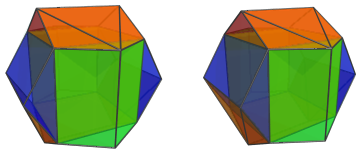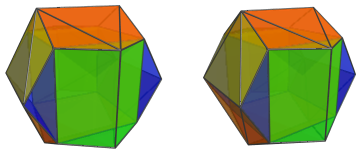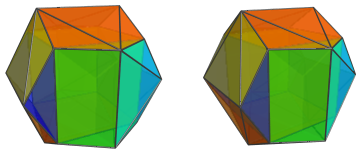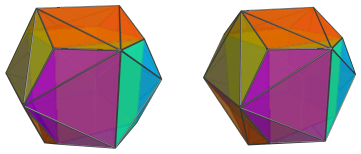These square pyramids look quite distorted because they lie at an angle to the 4D viewpoint, and moreover in a rotated orientation. Nevertheless, they are perfectly CRF square pyramids in 4D.

These are all the cells that lie on the near side of the polychoron. The remaining cells lie on the far side, that is, facing away from the 4D viewpoint.

### The Far Side

On the far side, of course, lies the icosahedron, which is antipodal to the cube.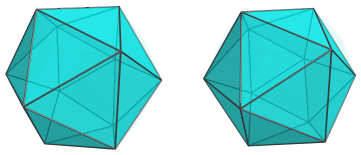Eight of this icosahedron's faces are joined to 8 tetrahedra: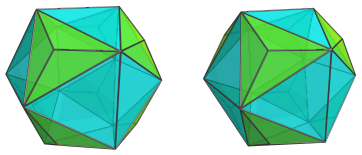These tetrahedra look flattened due to their angle with the 4D viewpoint; but they are, of course, perfectly regular tetrahedra in 4D.

These are all the cells that lie on the far side of the polytope. The rest of the icosahedron's faces are joined to the square pyramids that we have seen on the near side.

### Summary

The following table summarizes the cell counts for this polytope:

Region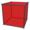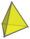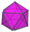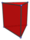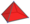Near side 1 0 0 6 12
Far side 0 8 1 0 0
Grand total 28 cells

## Coordinates

The Cartesian coordinates of K4.21, with edge length 2, are:

• (0, ±1, ±φ, 0)
• (±1, ±φ, 0, 0)
• (±φ, 0, ±1, 0)
• (±1, ±1, ±1, φ)

where φ=(1+√5)/2 is the Golden Ratio.

Last updated 18 Jun 2019.